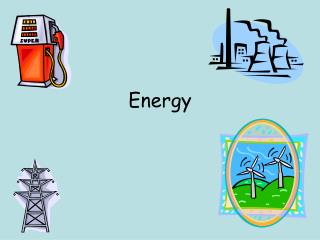# Energy - PowerPoint PPT PresentationDownload PresentationEnergy

EnergyDownload Presentation## Energy

- - - - - - - - - - - - - - - - - - - - - - - - - - - E N D - - - - - - - - - - - - - - - - - - - - - - - - - - -
##### Presentation Transcript

1. Energy

2. Energy & Work • Closely related • Energy: ability to do work • Work: transfer of energy • Both measured in Joules

3. Energy Types • Kinetic Energy: Energy in motion • Potential Energy:Stored energy (not moving) • depends on position or condition of object

4. Examples of KE

5. Examples of PE Boulder on top of cliff Firecracker battery

6. Calculating KE KE = ½ mv2 m = mass v = velocity Which increases kinetic energy more: doubling the mass or doubling the velocity?

7. Gravitational PE • PE that depends on object’s height, and weight G.P.E. = mgh m = mass g = 9.8 m/s2 h= height. Which will have greater GPE: a rock on a 3 story ledge or a rock on a 20 story ledge?

8. Mechanical Energy • PE Converts to KE and vice versa • Mechanical Energy = KE + PE • Total energy • Skier • Roller Coaster • Dart

9. Energy conversions • Law of conservation of energy: • Energy cannot be created or destroyed, only converted from one form to another